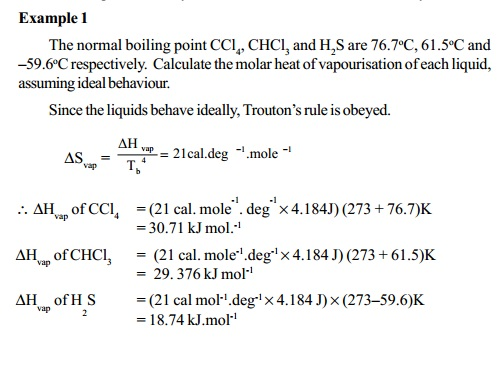Home | | Chemistry | Entropy And Entropy Change

# Entropy And Entropy Change

The entropy function 'S' introduced in the second law of thermodynamics is explained as below. Entropy function 'S' represents the ratio of the heat involved (q) to the temperature (T) of the process. That is S = q/T . This relation is valid only for reversible processes.

ENTROPY AND ENTROPY CHANGE

The entropy function 'S' introduced in the second law of thermodynamics is explained as below.

Entropy function 'S' represents the ratio of the heat involved (q) to the temperature (T) of the process. That is S = q/T . This relation is valid only for reversible processes. If a system is changed from state 1 to state 2 at constant temperature and if dq rev is the heat involved in the process, then entropy change of the process (DS) is given by,

S 2 - S1 = D S

= �12  (dq rev / T)

where the process is a reversible one. Entropy (S) and the change in entropy of the process (DS) are each state functions even though q and dq path functions.

In a reversible process, entropy of universe remains a constant.

S universe = S system + S surroundings =  Constant

Entropy change (DS) can be derived for various thermodynamic processes as below:

Isothermal process (T = constant)

DS = 12  (dq rev / T) = (q2 - q1 ) / T

Isothermal and isobaric process (T and P = Constant)

12  (dq P, rev / T)

Isothermal, and isochoric process (T and V- Constant)

12  (dq V, rev  / T)

The term 'natural process means that the process is spontaneous and does not need to be induced. In order to find out whether a process is spontaneous or not, the entropy changes of the system and the surroundings for the stipulated process is considered. If this change is positive i.e. if the entropy of the universe increases, the process will take place spontaneously and irreversibly.

If the entropy change of the universe is zero or negative (DS<0) the system will behave as non spontaneous.

When the external pressure is less than the internal pressure of a gaseous system, the gas expands spontaneously. When volume increases in expansion, the disorder in the movement of gaseous molecules increases. Thus, spontaneous processes are associated with increase in disorder. When disorder in a process is favoured it occurs spontaneously and we say, the entropy change is positive. Entropy is a measure of microscopic disorder in the system and also represents spontaneity.

For a reversible process,

DS system = - DS surroundings

DSuniverse= DS system + DS surroundings = 0.

For an irreversible (spontaneous) process.

DSuniv> 0 (positive value)

Entopy change in a physical (phase) transformation can be determined particularly for evaporation of liquids at the boiling points, using Trouton's rule.According to this rule, the heat of vaporisation (DHvap)in calories per mole divided  by the boiling point of the liquid in Kelvin is a constant equal to 21 cal deg-1 mole-1 and is known as the entropy of vapourisaiton.

DSvap  = 21cal.deg-1mole-1

DHvap = Enthalpy change of vapourisation = Latent heat of vapourisation.

This equation is useful for estimating the molar heat of vaporization of a liquid of known boiling point. Substances that deviate from this rule are as follows:

i) Low boiling liquids such as hydrogen and Helium which boil only a little above 0K.

ii) Polar substances like water, alcohol which form hydrogen bonded liquids and exhibit very high boiling points as well as high DHvap.

iii) Liquids such as acetic acid whose molecules are partially associated in the vapor phase and possess very low entropy vaporization which is very much less than 21 cals/ mol/deg. Those liquids that obey Troutons rule are said to behave ideally.Study Material, Lecturing Notes, Assignment, Reference, Wiki description explanation, brief detail
11th 12th std standard Class Organic Inorganic Physical Chemistry Higher secondary school College Notes : Entropy And Entropy Change |